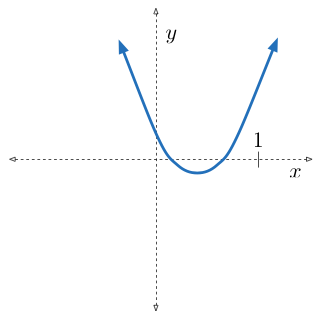# A Small Restriction With A Huge Impact

Algebra Level 4Positive integers $a, b$ and $c$ satisfy the condition that the quadratic equation $ax^2 - bx +c =0$ has 2 distinct real roots that are strictly between 0 and 1. What is the minimum value of $a$?

×